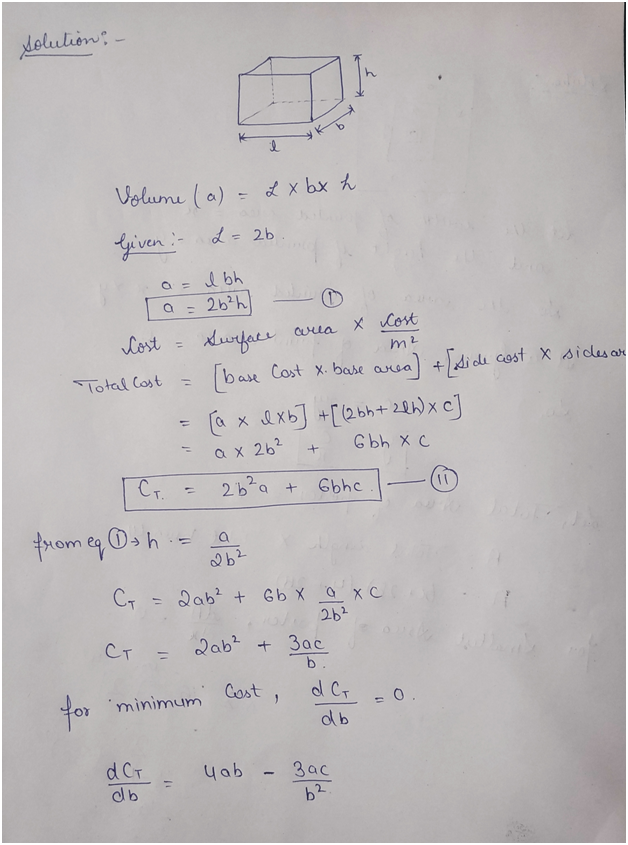# A rectangular storage container with an open top is to have a volume of a cubic meters. The length of its base is twice the width. Material for the base costs a dollars per square meter. Material for the sides costs c dollars per square meter. Find the cost of materials for the cheapest such container.Minimum cost =

Question
6 views

A rectangular storage container with an open top is to have a volume of a cubic meters. The length of its base is twice the width. Material for the base costs a dollars per square meter. Material for the sides costs c dollars per square meter. Find the cost of materials for the cheapest such container.

Minimum cost =

check_circle

Step 1

First draw the figure accordingly and solve:...

### Want to see the full answer?

See Solution

#### Want to see this answer and more?

Solutions are written by subject experts who are available 24/7. Questions are typically answered within 1 hour.*

See Solution
*Response times may vary by subject and question.
Tagged in